# Python Learning Week 11 (Solution)

Mini Project: Adversarial Search or Playing Games with Python

Game name: Tic Tac Toe (Using: The Minimax Algorithm)

PROJECT REPORT

TIC TAC TOE IN PYTHON

In this project we have created TIC TAC TOE game using minimax algorithm in
python.

The game is created using Tkinter which is a standard GUI library for python.
Tkinter provides a fast and easy way to create GUI applications. It provides
various controls, such as buttons, labels and text boxes used in a GUI application.
To install tkinter in Pycharm,
Go to File -> Settings(ctrl + alt + s) -> Project Interpreter -> click on “+” -> Search
for the Package name (Tkinter) -> Click on “Install Package”.
Consider the following diagram, it shows how an application actually executes
in Tkinter: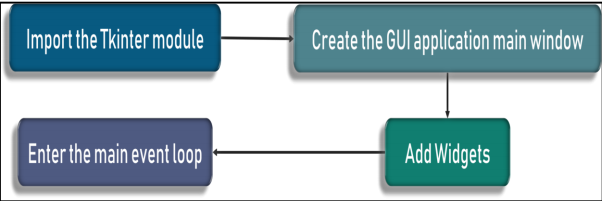To start out with, we first import the Tkinter model. Followed by that, we create
the main window. In this window we perform operations and display visuals.
Later, we add the widgets and lastly we enter the main event loop.

Commonly used Tkinter widgets are: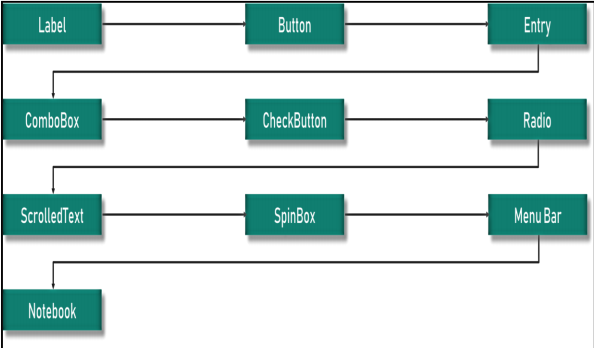Games are a form of competitive multi-agent environments where other agents
are playing against us. The purpose of designing game-playing programs is to
better understand how to choose actions in complex domains with uncertain
outcomes. In this project two players are used. X as (MAX player) and O as (MIN
player). The board consist of nine entries. MAX (X) takes the first move. After
every movement of X the value of opponent (O) is updated automatically on the
board. Every movement of (O) is optimal which means X has to take optimal
decisions otherwise X will lose the game. If X lose, the game will stop
automatically. Reset button is also used to replay the game.

CODE:

from tkinter import Tk, Button

from copy import deepcopy # By using deepcopy any changes made to a copy of object donot reflect in orignal object.

from tkfontchooser import Font

class disp:

# defining players, areas and size.

def __init__(self, other=None):

self.player = ‘X’

self.opponent = ‘O’

self.empty = ”

self.size = 3

self.areas = {}

for k in range(self.size):

for h in range(self.size):

self.areas[h, k] = self.empty

if other:

self.__dict__ = deepcopy(other.__dict__)

# defining movement of players

def move(self, h, k):

board = disp(self)

board.areas[h, k] = board.player

(board.player, board.opponent) = (board.opponent, board.player)

return board

# defining possible conditions using minimax

def __minimax(self, player):

if self.won():

if player:

return (-1, None)

else:

return (+1, None)

elif self.tied():

return (0, None)

elif player:

best = (-2, None)

for h, k in self.areas:

if self.areas[h, k] == self.empty:

value = self.move(h, k).__minimax(not player)

if value > best:

best = (value, (h, k))

return best

else:

best = (+2, None)

for h, k in self.areas:

if self.areas[h, k] == self.empty:

value = self.move(h, k).__minimax(not player)

if value < best:

best = (value, (h, k))

return best

def best(self):

return self.__minimax(True)

def tied(self):

for (h, k) in self.areas:

if self.areas[h, k] == self.empty:

return False

return True

def won(self):

####for horizontal values

for k in range(self.size):

winning = []

for h in range(self.size):

if self.areas[h, k] == self.opponent:

winning.append((h, k))

if len(winning) == self.size:

return winning

####for vertical values

for h in range(self.size):

winning = []

for k in range(self.size):

if self.areas[h, k] == self.opponent:

winning.append((h, k))

if len(winning) == self.size:

return winning

####for diagonal values

winning = []

for k in range(self.size):

h = k

if self.areas[h, k] == self.opponent:

winning.append((h, k))

if len(winning) == self.size:

return winning

####for other diagonal values

winning = []

for k in range(self.size):

h = self.size – 1 – k

if self.areas[h, k] == self.opponent:

winning.append((h, k))

if len(winning) == self.size:

return winning

####for default

return None

def __str__(self):

string = ”

for k in range(self.size):

for h in range(self.size):

string += self.areas[h, k]

string += “\n”

return string

class interface:

# defining interface of board (title, font,color and size)

def __init__(self):

self.app = Tk()

self.app.title(‘TIC TAC TOE’)

self.app.resizable(width=False, height=False)

self.board = disp()

self.font = Font(family=”Arial”, size=50,)

self.buttons = {}

for h, k in self.board.areas:

handler = lambda h=h, k=k: self.move(h, k)

button = Button(self.app, command=handler, font=self.font, width=4, height=1, bg=”yellow”)

button.grid(row=k, column=h)

self.buttons[h, k] = button

handler = lambda: self.reset()

button = Button(self.app, text=’RESET’,bg=”brown”, command=handler, fg=”White”)

button.grid(row=self.board.size + 1, column=0, columnspan=self.board.size, sticky=”S”)

self.update()

# to reset board in case of draw or win and if you want to play again

def reset(self):

self.board = disp()

self.update()

def move(self, h, k):

self.app.config(cursor=”watch”)

self.app.update()

self.board = self.board.move(h, k)

self.update()

move = self.board.best()

if move:

self.board = self.board.move(*move)

self.update()

self.app.config(cursor=””)

# for updating value after every movement of X

def update(self):

for (h, k) in self.board.areas:

text = self.board.areas[h, k]

self.buttons[h, k][‘text’] = text

self.buttons[h, k][‘disabledforeground’] = ‘blue’

if text == self.board.empty:

self.buttons[h, k][‘state’] = ‘normal’

else:

self.buttons[h, k][‘state’] = ‘disabled’

winning = self.board.won()

if winning:

for h, k in winning:

self.buttons[h, k][‘disabledforeground’] = ‘black’

for h, k in self.buttons:

self.buttons[h, k][‘state’] = ‘disabled’

for (h, k) in self.board.areas:

self.buttons[h, k].update()

# using of main loop to wait for an event to occur and process that event as long as window is not closed.

def mainloop(self):

self.app.mainloop()

if __name__ == ‘__main__’:

interface().mainloop()

OUTPUT: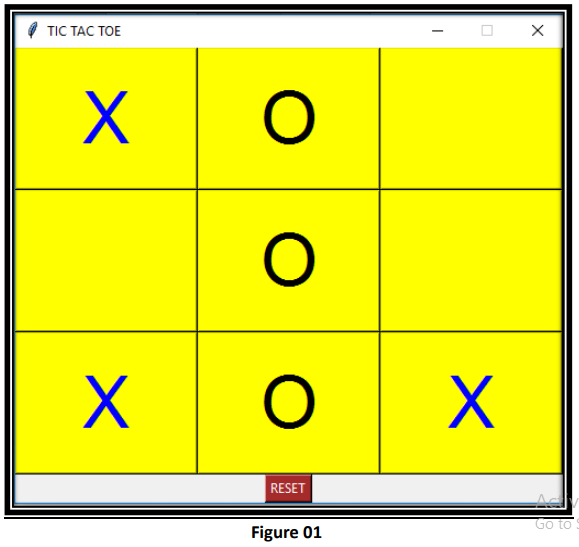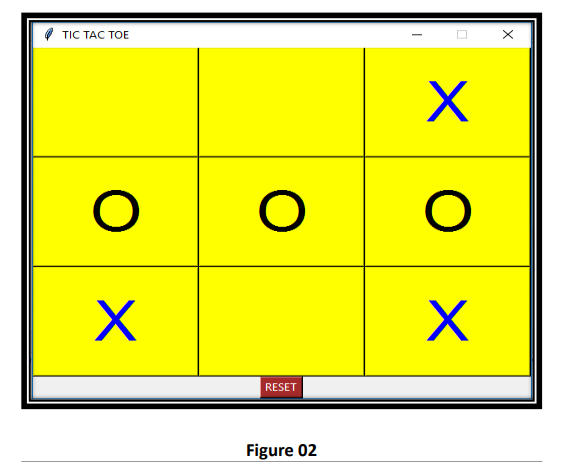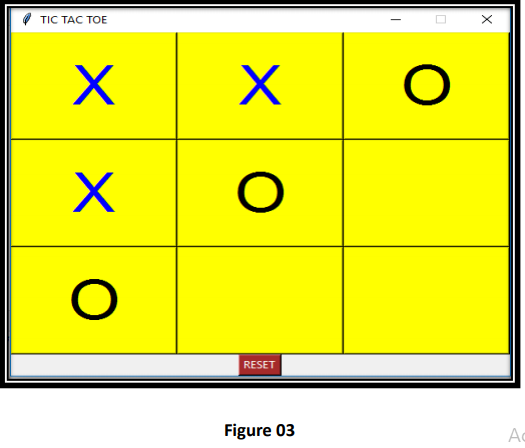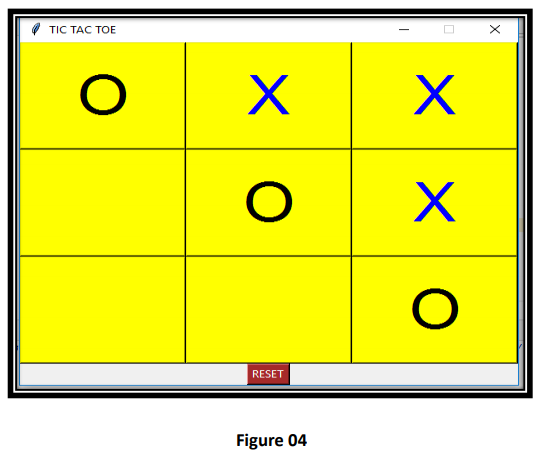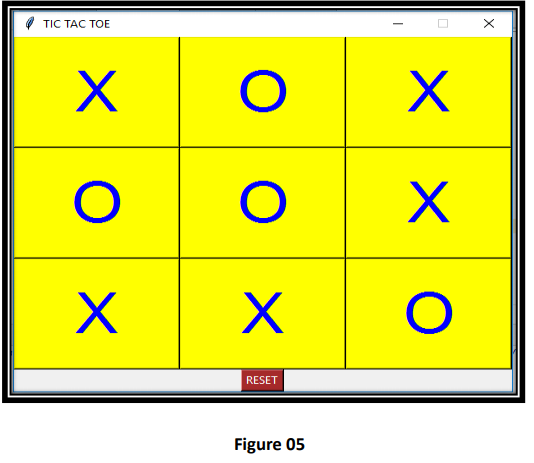CONCLUSION:

In this project we understand how to implement games in python. By using minimax algorithm and tkinter we build TIC TAC TOE game. Tkinter is a standard python interface for building GUI applications. We create a 3×3 board and define possible moves and conditions for players and also use a reset button to replay the game. After that we select font and color of title and board and by executing code we get the output.